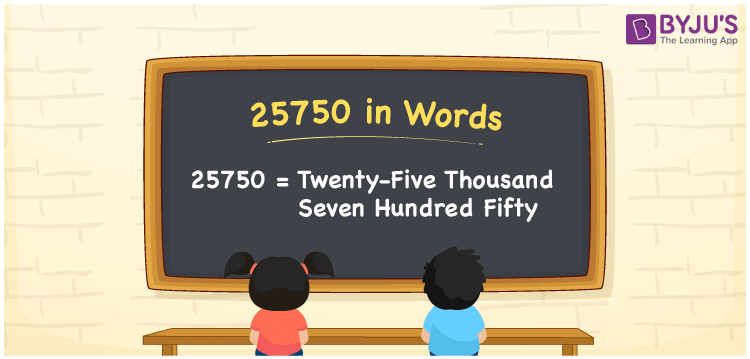25750 in words is written as Twenty Five Thousand Seven Hundred and Fifty. In 25750, 2 has a place value of ten thousand, first 5 has the place value of thousand, 7 is in the place value of hundred and next 5 is in the place value of ten. The article on Place Value gives more information. The number 25750 is used in expressions that relate to money, distance, length, social media views, and many more. For example, “A twitter campaign had Twenty Five Thousand Seven Hundred and Fifty tweets in the first one hour.”

 25750 in words Twenty Five Thousand Seven Hundred and Fifty Twenty Five Thousand Seven Hundred and Fifty in Numbers 25750

## 25750 in English Words## How to Write 25750 in Words?

We can convert 25750 to words using a place value chart. This can be done as follows. The number 25750 has 5 digits, so let’s make a chart that shows the place value up to 5 digits.

 Ten thousand Thousands Hundreds Tens Ones 2 5 7 5 0

Thus, we can write the expanded form as:

2 × Ten thousand + 5 × Thousand + 7 × Hundred + 5 × Ten + 0 × One

= 2 × 10000 + 5 × 1000 + 7 × 100 + 5 × 10 + 0 × 1

= 25750.

= Twenty Five Thousand Seven Hundred and Fifty.

25750 is the natural number that is succeeded by 25749 and preceded by 25751.

25750 in words – Twenty Five Thousand Seven Hundred and Fifty.

Is 25750 an odd number? – No.

Is 25750 an even number? – Yes.

Is 25750 a perfect square number? – No.

Is 25750 a perfect cube number? – No.

Is 25750 a prime number? – No.

Is 25750 a composite number? – Yes.

## Solved Example

1. Write the number 25750 in expanded form

Solution: 2 x 10000 + 5 x 1000 + 7 x 100 + 5 x 10 + 0 x 1

Or Just 2 x 10000 + 5 x 1000 + 7 x 100 + 5 x 10

We can write 25750 = 20000 + 5000 + 700 + 50 + 0

= 2 x 10000 + 5 x 1000 + 7 x 100 + 5 x 10 + 0 x 1.

## Frequently Asked Questions on 25750 in words

### How to write the number 25750 in words?

25750 in words is written as Twenty Five Thousand Seven Hundred and Fifty.

### Is 25750 divisible by 3?

No. 25750 is not divisible by 3.

### Is 25750 a prime number?

No. 25750 is not a prime number.